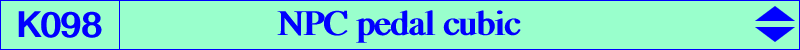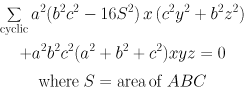vertices of the circum-tangential triangle infinite points of K024 feet of the trilinear polar of X(1994), the orthocorrespondent of X(5)In general, the locus of point M whose pedal circle passes through a fixed point Q is an isogonal nK whose root is the orthocorrespondent of Q (see "Special isocubics" §7.2.4 and "Orthocorrespondence..." in the Downloads page). These cubics form the class CL036. This nK is a nK60 if and only if Q = X(5) = nine point center. The cubic is called K098 or NPC pedal cubic. Its asymptotes are parallel to the Morley triangle sidelines. Their common points form an equilateral triangle centered at X(2) whose circumradius is R/3. The root is X(1994), orthocorrespondent of X(5), the nine point center. K098 is a member of the class CL004 of cubics. See a generalization in the page K687.